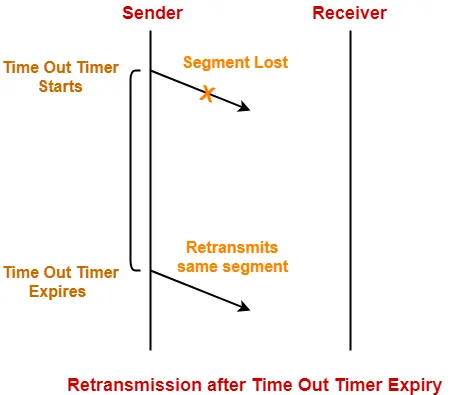# Transmission Control Protocol | Practice Problems

## Problem-01:

How many TCP connections can be opened between two ports?

1. Multiple
2. Single
3. Zero
4. None

## Solution-

Option (B) is correct.

## Problem-02:

TCP protects itself from miss delivery by IP with the help of-

1. Source IP Address in IP header
2. Destination IP Address in IP header
4. Source port and Destination port

## Solution-

Option (C) is correct.

## Problem-03:

What addressing system has topological significance?

1. Logical or Network Address
2. LAN or Physical Address
3. Port Addressing System
4. Multicast Addressing System

## Solution-

Option (A) is correct.

## Problem-04:

If WAN link is 2 Mbps and RTT between source and destination is 300 msec, what would be the optimal TCP window size needed to fully utilize the line?

1. 60,000 bits
2. 75,000 bytes
3. 75,000 bits
4. 60,000 bytes

## Solution-

Given-

• Bandwidth = 2 Mbps
• RTT = 300 msec

### Optimal TCP Window Size-

Optimal TCP window size

= Maximum amount of data that can be sent in 1 RTT

= 2 Mbps x 300 msec

= 600 x 103 bits

= 60,0000 bits

= 75,000 bytes

Thus, Option (B) is correct.

## Problem-05:

Suppose host A is sending a large file to host B over a TCP connection. The two end hosts are 10 msec apart (20 msec RTT) connected by a 1 Gbps link. Assume that they are using a packet size of 1000 bytes to transmit the file. For simplicity, ignore ack packets. At least how big would the window size (in packets) have to be for the channel utilization to be greater than 80%?

1. 1000
2. 1500
3. 2000
4. 2500

## Solution-

Given-

• RTT = 20 msec
• Bandwidth = 1 Gbps
• Packet size = 1000 bytes
• Efficiency >= 80%

### Window Size For 100% Efficiency-

For 100% efficiency,

Window size

= Maximum number of bits that can be transmitted in 1 RTT

= 1 Gbps x 20 msec

= (109 bits per sec) x 20 x 10-3 sec

= 20 x 106 bits

= 2 x 107 bits

### Window Size For 80% Efficiency-

For 80% efficiency,

Window size

= 0.8 x 2 x 107 bits

= 1.6 x 107 bits

In terms of packets,

Window size

= 1.6 x 107 bits / Packet size

= 1.6 x 107 bits / (1000 x 8 bits)

= 0.2 x 104 packets

= 2000 packets

Thus, Option (C) is correct.

## Problem-06:

A TCP machine is sending windows of 65535 B over a 1 Gbps channel that has a 10 msec one way delay.

1. What is the maximum throughput achievable?
2. What is the line efficiency?

## Solution-

Given-

• Window size = 65535 bytes
• Bandwidth = 1 Gbps
• One way delay = 10 msec

### Method-01:

Maximum amount of data that can be sent in 1 RTT

= 1 Gbps x (2 x 10 msec)

= (109 bits per sec) x 20 x 10-3 sec

= 20 x 106 bits

= 25 x 105 bytes

Amount of data that is actually being sent in 1 RTT = 65535 bytes

Thus,

Line Efficiency(η)

= Amount of data being sent in 1 RTT / Maximum amount of data that can be sent in 1 RTT

= 65535 bytes / 25 x 105 bytes

= 0.026214

= 2.62%

Now,

Maximum Achievable Throughput

= Efficiency x Bandwidth

= 0.0262 x 1 Gbps

= 26.214 Mbps

### Method-02:

Maximum Achievable Throughput

= Number of bits sent per second

= 65535 B / 20 msec

= (65535 x 8 bits) / (20 x 10-3 sec)

= 26.214 Mbps

Now,

Line Efficiency

= Throughput / Bandwidth

= 26.214 Mbps / 1 Gbps

= 26.214 x 10-3

= 0.026214

= 2.62%

Next Article- TCP Congestion Control

Get more notes and other study material of Computer Networks.

Watch video lectures by visiting our YouTube channel LearnVidFun.

SummaryArticle Name
Transmission Control Protocol | Practice Problems
Description
Practice Problems based on Transmission Control Protocol. TCP Protocol is a transport layer protocol. TCP header format specifies various fields required for transmission. TCP congestion control policy is used for handling congestion.
Author
Publisher Name
Gate Vidyalay
Publisher Logo